Published: 31 March 2015

# Influences of planetary gear parameters on the dynamic characteristics – a review

Feng Li1
Yumo Qin2
Linshan Ge3
Zhao Pang4
Shaokang Liu5
Donglong Lin6
1, 2, 3, 4, 5, 6College of Mechanical Science and Engineering, Jilin University, Changchun, 130022, China
Corresponding Author:
Yumo Qin
Views 699

#### Abstract

Planetary gear trains (PGTs) are widely used in the field of mechanical transmission. PGTs significantly differ from fixed-axis gear trains and exhibit unique dynamic behavior. Dynamic characteristics of PGTs are popular research topic, particularly when attempting to solve the problem of vibration noise. Moreover, the effects of the planetary gear parameters on the dynamic characteristics are paramount important. And significant researches have been conducted in this field. However, few reviews regarding these studies have been published. In this paper, the effects of certain parameters, which include mesh phase difference, geometric errors (tooth profile error, eccentricity error and misalignment), tooth profile modification, mesh stiffness, and etc., on the dynamic characteristics of PGTs are summarized. Several conclusions obtained can be used for the PGTs design and dynamic characteristics analysis. Finally, the potential research trends are pointed out.

## 1. Introduction

PGTs are widely used in aerospace, automotive transmission, wind turbine and heavy industry applications and so on, owning to their high efficiency level, large transmission ratio and small volume, etc. . Fig. 1 shows an example PGT has a ring gear, a sun gear that rotates around its own center. The planet gears are connected by bearings to the carrier and are simultaneously in mesh with both of the sun gear and ring gear. So the planet gears that not only rotate around the axis of carrier but also revolve around the center of the sun gear. The power is passed by the planet gears. The number of the planet gears is depended on the load of the PGT . PGTs can achieve higher power density levels, because they use multiple parallel power paths formed by individual planet branches, and this coaxial and axisymmetric nature of PGTs makes them more compact .

Fig. 1Schematic diagram of PGT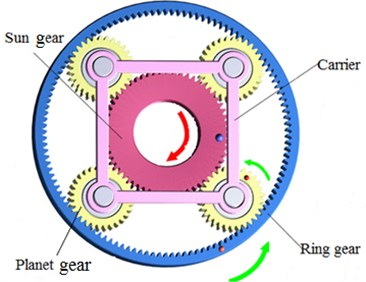However, PGTs vibration and noise problems  are primary concerns in these applications because of the complexity of their system structures. But the vibration of the system can lead to excessive dynamic load and noise. The dynamic forces at the sun-planet and ring-planet meshes increase the risk of tooth or bearing failures. And the noise, which is caused by the vibration of gears, is important factor influencing occupant comfort and a measure of vehicle quality. Vibration can cause structural failure. These problems affect the transmission efficiency and service life of PGTs. The dynamic characteristics of PGTs are primary concerns both in terms of vibration and noise. In order to reduce vibration and noise of PGT, dynamics analysis is indispensable. On the other hand, PGTs significantly differ from fixed-axis gearboxes and exhibit unique dynamic behavior resulting in added noise, vibration, acoustic emissions, and unacceptable performance characteristics. These questions are severest when the transmission works in poor conditions (profile errors on teeth, geometric errors or lacking stiffness, etc.). Therefore, understanding the effects of these parameters on the dynamic behavior of PGTs is useful in design and analysis.

A number of papers have been published on dynamic characteristics of PGT, which consist of a finite element model [5-7] and deformable or hybrid models of varying complexities [8, 9]. Bu et al.  and Wu S. J. et al.  have reviewed the analysis model type of planetary gears, dynamic characteristics, calculation method of the dynamic respond, and so on. Meanwhile, the planetary gear dynamic characteristics are also affected by the parameters of the PGT. Sondkar and Kahraman  proposed a dynamic model of a double-helical PGT to demonstrate the effect of key design parameters including the right-to-left stagger angle, and support conditions on the dynamic response of the PGT. Parker and Lin  clarified the mesh phase properties for general planetary gears and provide a complete analytical description in terms of the fundamental parameters of tooth numbers and planet locations. Some analyses of the effects of mesh phase on planetary gear dynamic characteristics were conducted [14-21]. Several studies [22-24] confirmed that tooth profile errors can cause incorrect gear mesh and affect the transmission characteristic of the PGT.

The main research contents of this paper are as follows: in the first part, the effects of planetary gear parameters on the dynamics characteristics are summarized, which mainly include the mesh phase difference, geometric errors (tooth profile errors, electricity error and misalignment), TPM, and mesh stiffness. In the second part, the conclusions are discussed and summarized. These conclusions can be used to guide dynamic characteristic analysis and planetary gear design. Finally, future research trends in this area are described.

## 2. Effects of planetary gear parameters on dynamic characteristics

The effects of the planetary gear parameters on the dynamic characteristics are important to obtain an excellent PGT design. In the next part, the effects of the major planetary gear parameters on dynamic characteristic are respectively reviewed.

### 2.1. Mesh phase difference

Substantial researches [13-15, 18, 25] have been conducted on the mesh phase of PGT, which show that a mapping relationship exists between the phase matching and the excitation of PGT.

Jiang studied the effect of the mesh phase of PGT on gear vibration and noise to better understand the application of mesh phase difference on the PGT. This application can reduce the gear vibration and noise levels, and these levels were less susceptible to the influence of the tooth surface mesh coefficient  and the tooth profile contact ratio [19, 26]. Chen and Ishibashi  designed and built an original PGT testing machine to examine how vibration and noise were affected by different contact ratios and meshing phase difference, and the testing results shown that the noise and vibration levels of PGT with a meshing phase difference can be further reduced by improving gear accuracy and tooth face roughness. Meanwhile, the PGT exists with combination of various mesh stiffness because of the mesh phase difference. This condition may lead to the PGT appearing to have a more dense frequency , and create a large difference for each planet gear vibration mode and an uneven load distribution. Thus, the reliability of PGT is reduced.

In the PGT, different mesh phase between the sun-planet and ring-planet mesh has powerful impact on the dynamic response and can have significant benefits in reducing vibration and noise[18, 19]. So a clear understanding of the relations governing the mesh phase is essential. Parker and Lin  clarified the mathematical relations for mesh phase for general PGT.

In the PGT, the planet gears are evenly distributed around the sun gear and each planet gear has the same parameters, the condition results in the periodic time-varying mesh stiffness of the sun gear. Through the design of the mesh phase difference, each planet gear acquires a different mesh phase and the effects of the excitation caused by mesh stiffness  can be reduced after superposition. Finally, the vibration can be neutralized or eliminated . As shown in Fig. 2, a reasonable mesh phase difference was designed in PGT. Although the time-varying stiffness of each planet gear varies with the mesh periodic, the total mesh stiffness of PGT is a fixed value after the superposition.

Fig. 2Mesh stiffness of PGT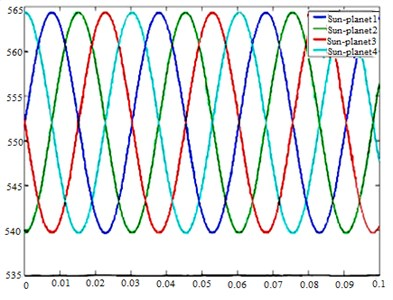a) Instantaneous mesh stiffness of sun-planets mesh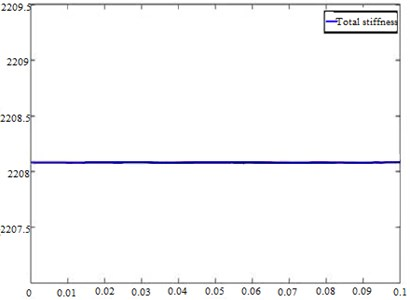b) Total mesh stiffness of PGT after superposition

Therefore, the ultimate goal of designing the mesh phase difference is to change the time-varying mesh stiffness of the PGT. Finally, the vibration and noise are reduced.

### 2.2. Geometric errors

The effects of the geometric errors on the PGT dynamic characteristics have been studied extensively. Cheon and Parker  and James  studied the effect of manufacturing errors on the PGT dynamic characteristics. Typical errors of manufacturing errors, which include modulations include eccentricities and run-out errors of the gears and the carrier, and tooth-to-tooth spacing, tooth thickness and so forth, might cause modulations of PGT . The combination of a series of incentive disturbance, which caused by the tooth profile errors, mounting errors, and deflections , cause the transmission error of PGT so that the vibration and noise are produced. The effects of the tooth profile errors, the eccentricities and the misalignments on the dynamic characteristics of the PGT are summarized in the follow paragraphs.

### 2.2.1. Tooth profile errors

Generally, tooth profile errors, which are induced by the manufacturing process, result in imperfections of the geometry of the tooth and create deviations from the perfect involute shape [31, 32]. As shown in Fig. 3, the solid lines show the actual tooth profile shapes and the dashed lines indicate the two closest tooth profile shape lines containing the actual tooth profile shape line.

Fig. 3Tooth profile errors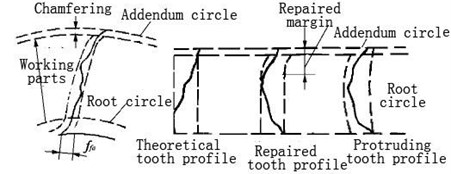The tooth profile errors may affect the contact quality of the tooth surface and cause the incorrect gear mesh, finally affect the transmission characteristic of the PGT. Moreover, the tooth profile errors can increase the gear mesh frequency amplitude. High values of these errors indicate poorly manufactured gears and tend to have dramatic consequences on the transmission . A tooth profile can product an exciting force $F\left(t\right)$, which represents an amplitude modulation of the gear mesh stiffness signal ${K}_{e}\left(t\right)$ by the profile error signal ${E}_{sn}\left(t\right)$. It is expressed by:

1
$F\left(t\right)={E}_{sn}\left(t\right){K}_{e}\left(t\right),$
2
${E}_{sn}\left(t\right)={\sum }_{m=1}^{\mathrm{\infty }}{\stackrel{-}{E}}_{sm}\mathrm{s}\mathrm{i}\mathrm{n}\left(2\pi m{f}_{e}t+{\xi }_{sn}\right),$

where ${\stackrel{-}{E}}_{sm}$ represents the profile error modulus, ${f}_{e}$ is the mesh frequency, ${\xi }_{sn}$ is the phase difference of sun-planets mesh and $m$ is the multiplicity of eigenvalues.

A previous study  suggested the tooth profile errors might be the cause of these variations in rattle amplitude. Another study  concluded that the composite profile errors of a gear pair had a stronger effect on the gear noise than the profile errors of a single gear. Moreover, the vibration noise of the gear mesh is correlated not only with the size of the shape errors but also with the direction of the tooth profile pressure angle error. At the meantime, the dynamic characteristics of PGT are affected by the tooth profile errors through affecting the mesh phase.

### 2.2.2. Eccentricity error

Eccentricity may be introduced during manufacture, or during set-up via shaft misalignment, imperfections in grub screw (set screw) tightness or ill- fitting bearings . Moreover, eccentricity errors generate dynamic excitations and complex modulations which may downgrade the overall dynamic performance. To classic parallel axis gears, PTGs can be very sensitive to eccentricities, even in static conditions, when no central member is allowed to float. Chaari et al.  studied the effects of the eccentricity error (run-out error) on PGT dynamic response. He concluded that an exciting force is produced by the eccentricity error, which is expressed by Eq. (3), (4):

3
$F\left(t\right)={e}_{sn}\left(t\right){K}_{e}\left(t\right),$
4
${e}_{sn}\left(t\right)={\stackrel{-}{e}}_{s}\mathrm{s}\mathrm{i}\mathrm{n}\left(\frac{2\pi {f}_{e}}{{Ζ}_{s}}t+{\xi }_{sn}\right).$

Gu and Velex  concluded that the eccentricity error could lead to contact loss at the critical speed. As far as dynamic tooth loads are considered, eccentricity errors do not introduce additional critical speeds but lead to more complex response spectra with modulation sidebands. As shown in Fig. 4, the eccentricity error increases the gear load ratio, which causes the gear tooth to bear excessive dynamic load and eventually leads to transmission failure. Furthermore, the gear with eccentricity errors, which subjected the varying mesh stiffness, can produce larger load ratio than the gear subjected constant mesh stiffness.

Fig. 4Effect of eccentricity error on gear load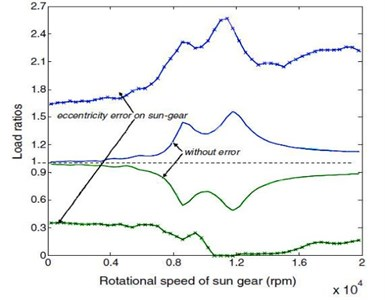a) Maximum and minimum tooth load ratio of sun/planet gearb) Maximum load ratio of sun/planet gear with different eccentricity error

### 2.2.3. Misalignment

The misalignment of PGT varies with time, which results in increasing the vibration, the time-varying contact stress and the probability of the failure of PGT, and reducing the gear life . Litvin  reminded that due to misalignment the line contact of tooth surfaces is turned out into point contact. Litvin et al.  concluded that misalignment can cause the discontinuous transmission errors, and the bearing contact should be oriented longitudinally to increase the contact ratio. Thus the shift of bearing contact caused by misalignment is reduced. Crowther et al.  pointed out that when the bearing clearance is optimal, the contact stress amplitude can be decreased and a mean stress is achieved (Fig. 5). The gears are still misaligned, but this misalignment is now consistent with the rotation and can be corrected with lead slope adjustments.

Fig. 5Planet gears bearing maximum contact stress with carrier rotation after planet gears bearing clearance optimization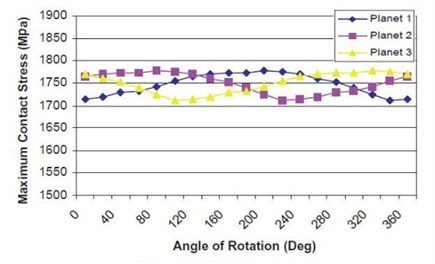Through the analysis of Crowther et al. , the misalignment is relative to bearing clearance of PGT, so the misalignment can be reduced by optimizing the bearing clearance.

Vecchiato  applied the new ideas of tooth contact analysis to prove that an isostatic PGT is a self-regulated system because of the existence of floating gears. He considered an isostatic planetary gear train is not sensitive to most misalignment and has capable of self-regulation in order to compensate for various alignment errors.

### 2.3. Tooth profile modification (TPM)

Excessive gear tooth deflection due to applied torque causes undesirable tooth contact patterns and increases gear vibration, noise, and contact stress. TPM is extensively used to compensate for the elastic gear and tooth deflection from the applied torque. Moreover, TPM can improve load distribution of PGT , eliminate the tooth elastic deformation, increase wear resistance and reduce gear contact stress. Meanwhile Abousleiman and Velex  showed that tip relief greatly reduces dynamic tooth loads and displacement amplitudes by the numerical simulations of the PGT model. Depending on the amount of mesh stiffness fluctuation and the mesh phase, different TPMs are required to minimize the gear vibration . The changing of the sun-planet mesh stiffness with regard to TPM is shown in Fig. 6.

Fig. 6Sun-planet mesh stiffness with TPM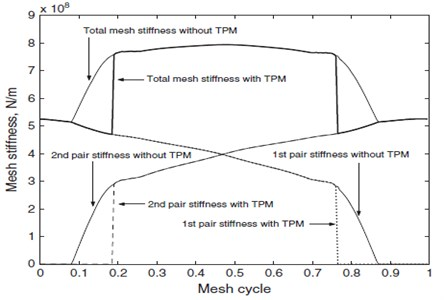TPM is used to optimize contact patterns, to compensate manufacturing errors, and to reduce gear dynamic destruction. Liu and Parker  concluded that for the multimesh gear trains, the dynamic effect of the TPM is commonly correlated with the static transmission error. Furthermore, the TPM of individual mesh gear is correlated with mesh stiffness variations and contact ratios. The gear contact pattern is mainly affected by the TPM and dynamic response [41, 42].

To analyze the actual effect of the TPM on vibration reduction, Wu Y. J. et al.  studied the vibration level reduction under different working conditions through an experiment, as illustrated in Fig. 7.

Fig. 7Experimental vibration reduction of TPM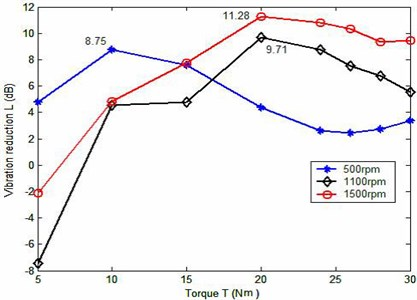Fig. 6 shows that the effect of the presented TPM is not independent with the working conditions. With the increase of the torque, the effect of the TPM on vibration reduction is different for each speed, but the trends of the vibration reduction are almost the same for different speeds.

The TPM may deviate from the optimal area along with the change of mesh phase during optimization, and considering the interaction between the two gears mesh is necessary. According to Liu and Parker ,the TPM for the minimal static transmission error (STE) depends only on the load and mesh stiffness of the single mesh. The optimal TPM for the minimal dynamic transmission error (DTE) is determined by mesh stiffness variations, contact ratios, mesh phase, and vibration modes. The TPM for the minimal STE does not necessarily lead to the minimal DTE, and it is proved by Bahk and Parker  by experimental verification.

### 2.4. Mesh stiffness

Gear mesh stiffness is a parametric excitation, and the effect of its dynamic excitation on gear dynamic characteristics is one of the main points of this study. According to Kahraman and Blankenship  and Chaari  mesh stiffness variation is one of the major sources of gear vibration. The time-varying mesh stiffness is a periodic function caused by the change in the number of contact tooth pairs and the contact positions of the gear teeth . Experiments demonstrated that the large amplitude vibration is induced by parametric instability wherein the gear mesh frequency equals twice the natural frequency (primary instability). Mesh stiffness directly affects the gear deformation and transmission error. Therefore, gear resonance excited by the harmonics of the transmission error fundamentally arises from the mesh stiffness variation [47, 48].

Liang et al.  stated that when to evaluate the mesh stiffness of PGT, the Hertzian contact stiffness, bending stiffness, axial compressive stiffness and shear stiffness should be considered. By studying the vibration response of the single-stage ear pair, Tian et al.  concluded that for the single-tooth-pair mesh duration, the total mesh stiffness is expressed as:

5
${k}_{t}=\frac{1}{\frac{1}{{k}_{h}}+\frac{1}{{k}_{b1}}+\frac{1}{{k}_{s1}}+\frac{1}{{k}_{a1}}+\frac{1}{{k}_{b2}}+\frac{1}{{k}_{s2}}+\frac{1}{{k}_{a2}}},$

where subscripts 1 and 2 represent the pinion and the corresponding gear, respectively, ${k}_{h}$ is the Hertzian contact stiffness and ${k}_{h}=\pi EL/4\left(1-{v}^{2}\right)$, ${k}_{b}$is the bending stiffness and:

$\frac{1}{{k}_{b}}={\int }_{-{\mathrm{\alpha }}_{1}}^{{\mathrm{\alpha }}_{2}}\frac{\left\{3{\left\{1+\mathrm{c}\mathrm{o}\mathrm{s}{\alpha }_{1}\left[\left({\alpha }_{2}-\alpha \right)\mathrm{s}\mathrm{i}\mathrm{n}\alpha -\mathrm{c}\mathrm{o}\mathrm{s}\alpha \right]\right\}}^{2}×\left({\alpha }_{2}-\alpha \right)\mathrm{c}\mathrm{o}\mathrm{s}\alpha \right\}}{2EL{\left[\mathrm{s}\mathrm{i}\mathrm{n}\alpha +\left({\alpha }_{2}-\alpha \right)\mathrm{c}\mathrm{o}\mathrm{s}\alpha \right]}^{3}}d\alpha ,$

${k}_{a}$ is the axial compressive stiffness and:

$\frac{1}{{k}_{a}}={\int }_{-{\alpha }_{1}}^{{\alpha }_{2}}\frac{\left({\alpha }_{2}-{\alpha }_{1}\right)\mathrm{c}\mathrm{o}\mathrm{s}\alpha \mathrm{s}\mathrm{i}{\mathrm{n}}^{2}{\alpha }_{1}}{2EL\left[\mathrm{s}\mathrm{i}\mathrm{n}\alpha +\left({\alpha }_{2}-\alpha \right)\mathrm{c}\mathrm{o}\mathrm{s}\alpha \right]}d\alpha ,$

${k}_{s}$ is the shear stiffness and:

$\frac{1}{{k}_{s}}={\int }_{-{\alpha }_{1}}^{{\alpha }_{2}}\frac{1.2\left(1+v\right)\left({\alpha }_{2}-{\alpha }_{1}\right)\mathrm{c}\mathrm{o}\mathrm{s}\alpha \mathrm{c}\mathrm{o}{\mathrm{s}}^{2}{\alpha }_{1}}{EL\left[\mathrm{s}\mathrm{i}\mathrm{n}\alpha +\left({\alpha }_{2}-\alpha \right)\mathrm{c}\mathrm{o}\mathrm{s}\alpha \right]}d\alpha ,$

${\alpha }_{2}$ is the half tooth angle on the base circle of the external gear, ${\alpha }_{1}$ is the angle between the force component ${F}_{b}$ and the acting force $F$ which can be decomposed into two orthogonal component forces: ${F}_{a}$ and ${F}_{b}$  (Fig. 8).

For the double-tooth-pair mesh duration, there are two pairs of gears mesh at the same time. The total mesh stiffness is expressed as :

6
${k}_{t}={\sum }_{i=1}^{2}\frac{1}{\frac{1}{{k}_{hi}}+\frac{1}{{k}_{b1i}}+\frac{1}{{k}_{s1i}}+\frac{1}{{k}_{a1i}}+\frac{1}{{k}_{b2i}}+\frac{1}{{k}_{s2i}}+\frac{1}{{k}_{a2i}}},$

where $i=$ 1 for the first pair of mesh teeth and $i=$2 for the second pair.

According to the above equations, ${F}_{spn}$ is the dynamic gear mesh forces which can be expressed as :

7
${F}_{spn}={k}_{spn}{\delta }_{spn}+{c}_{spn}{\stackrel{˙}{\delta }}_{spn},$

where ${k}_{spn}$ and ${c}_{spn}$ represents the $n$th sun-planet gear mesh stiffness and mesh damping, respectively, and ${\mathrm{\delta }}_{spn}$ denotes the compression of the nth sun-planet mesh spring.

Fig. 8Elastic force on the external gear tooth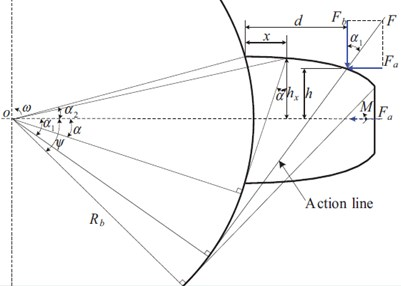The instability of the mesh stiffness of PGT is sensitive to the contact ratio and mesh phase, and the instability can be inhibited by changing the two parameters [51, 52]. Lin and Parker [51, 52] concluded that the parametric excitation from the time-varying mesh stiffness causes instability and severe vibration under certain operating conditions (that is, mesh frequency and stiffness variation amplitude).

### 2.5. Effects of other factors

In addition to the aforementioned parameters, the amount of planet gears , pressure angle , tooth width , clearances [56, 57], backlash  and bearing stiffness  also affect the dynamic behavior of the PGT.

For instance, the backlash, which is essential for assembly of the gears , namely the clearance, tends to make gear systems exhibit typical nonlinear dynamic behavior, undesirable noise and vibration problems [56, 61, 62]. Furthermore, with the increasing of the backlash, the tooth contact force is increased and the contact time is reduced (Fig. 7) . Litvin et al.  developed new types of PGT and the new designs were based on regulating backlash between the gears, which is to achieve almost uniform load distribution.

Fig. 9Gear contact force with different backlash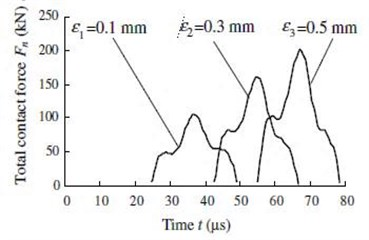## 3. Conclusions

PGTs may exhibit undesirable dynamic behavior resulting in added noise, acoustic emissions, and unacceptable performance characteristics. The studies of the effects of the parameters on the dynamic characteristics of PGT are essential. Based on the above summaries, several conclusions obtained can be used for PGT design and analysis of dynamic characteristics.

1. Mesh phase difference can reduce the effect of the tooth surface mesh coefficient on the vibration and noise. In the PGT, phase difference design is necessary to acquire a different mesh phase for each planet gear. Therefore, the effect of time-varying stiffness on the dynamic responses of PGT can be reduced after the superposition.

2. The contact quality of the tooth surface is affected by the tooth profile errors. The dynamic characteristic of PGT also is affected by the tooth profile errors through affecting the mesh phase. The composite profile errors of a gear pair is the key factor that affects gear noise.

3. The eccentricity error can lead to contact loss and more complex response spectra. Furthermore, the misalignment can cause discontinuous transmission errors. The misalignment of the PGT is sensitive to the size of the bearing clearance and the bearing contact. Therefore, the bearing clearance should be optimized firstly, and the bearing contact should be oriented longitudinally to reduce the negative effects of misalignment.

4. TPM can improve the load distribution, eliminate the tooth elastic deformation, increase wear resistance, and reduce the contact stress. Meanwhile, in optimizing the TPM for the minimum DTE, the interaction between the two meshes as well as the effect of mesh phase on TPM should be considered.

5. The gear resonance excited by the harmonics of the transmission error fundamentally arises from the mesh stiffness variation. The instability of the mesh stiffness parameters can be reduced by reasonably choosing the gear contact ratio and mesh phase. Furthermore, the effects of mesh stiffness on the vibration are relative to the operating conditions.

## 4. Research prospects

Several key areas that may be targeted in further research include:

1. The preceding summaries show that certain parameters have mutual effects, such as [41, 64]. Bahk and Parker  through the experiment to prove that dynamic response is not guaranteed to be minimized by TPM, instead it may continuously grow for larger TPM for certain mesh phase choices. Thus, it is critical to take into account the mesh phase when designing profile modifications for planetary gears. Comprehensively considering the mutual effect of the parameters is important to study the effect of the dynamic characteristics and design of the PGT.

2. In addition to the aforementioned factors, the oil film stiffness  should also be considered and the friction between the meshing teeth is inevitable and has been suggested to play a considerable role in gear dynamics [66, 67]. Furthermore, the effect of the parameters on the dynamic characteristics of the PGT should also be considered for the “lost oil” condition of the gear.

3. Significant researches have been conducted on the dynamic characteristics of single-stage PGT, but studies on the multi-stage PGTs are few . Thus, the mutual effect of compound planetary transmission [24, 69, 70] and the effects of various parameters on the dynamic characteristics of the compound PGTs require further research, such as that presented by the reference .

4. The effects of PGT parameters with non-circular gears  on dynamic characteristics should be studied further.

08 July 2014
Accepted
18 September 2014
Published
31 March 2015
SUBJECTS
Mechanical vibrations and applications
Keywords
planetary gear
parameters effects
dynamic characteristics
vibration and noise
review
Acknowledgements

The authors acknowledge the financial support from National Natural Science Foundation of China (Issue 51375202 and 51075179) and Scientific Frontier and Interdisciplinary Merit Aid Projects of Jilin University, China (Issue 2013ZY08).